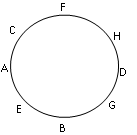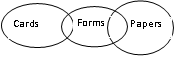# Ittiam Reasoning Question

DIRECTIONS for questions 1 to 5: Study the given information carefully and answer the given questions: Eight people -A, B, C, D, E, F, G and H are sitting around a circular table facing the centre, not necessarily in the same order. Three people are sitting between A and D. B is sitting second to the right of A. C is to the immediate right of F. D is not an immediate neighbour of either F or E. H is not an immediate neighbour of B.
1. What is E's position with respect to G?
1. Third to the left
2. Second to the right
3. Third to the right
4. Second to the left
5. Fifth to the rightFrom above figure it is clear that E is sitting 2nd to the left of G.
So, the correct answer is option D.
2. Four of the following five are alike in a certain way, based on their seating positions in the above arrangement and so form a group. Which one does not belong to the group?
1. GE
2. DC
3. AF
4. AB
5. CEFrom above figure it is clear that the answer is 2nd option, As the gap between the persons in all other pairs is of 1 person.
Therefore, the correct answer is option B.
1. Who is sitting third to the right of the one who is sitting to the immediate right of H?
1. A
2. B
3. E
4. C
5. GFrom above figure it is clear that answer is 3rd option.
2. Which of the following is "true" regarding the given arrangement ?
1. E is second to the left of C
2. B is an immediate neighbour of G
3. H is an immediate neighbour of A
4. D is not an immediate neighbour of H
5. None of theseFrom above figure it is clear that answer is 2nd option.
3. How many people are sitting between H and A when counted from the right side of H?
1. Three
2. None
3. More than three
4. One
5. TwoFrom above figure it is clear that answer is 5th option.
DIRECTIONS for questions 6 -7: The question below has either two or three statements followed by two or three conclusions. You have to take the given statements to be true and then decide which of the given conclusions logically follows from the given statements, disregarding the commonly known facts.
Give answer (1) : if only conclusion I follows
Give answer (2) : if only conclusion II follows
Give answer (3) : if either I or II follows
Give answer (4) : if neither I nor II follows
Give answer (5) : if both I and II follow
4. Statements:
i. Some forms are cards.
ii. Some forms are papers.
Conclusions:
I. Atleast some cards are forms.
II. Atleast some cards are papers.
1. 1
2. 2
3. 3
4. 4
5. 5I. This means atleast one card is form, which is definitely true for all the possible cases. So, conclusion I follows.
II. This means that atleast one card is paper,which is not a necessarily true answer.So, conclusion II does not follow.
Therefore, only conclusion 1 follows.So, the answer is option A.
5. Statements: i. All animals are predators. ii. All predators are tigers. Conclusions: I. All tigers are definitely predators. II. All animals are tigers.
1. 1
2. 1
3. 3
4. 4
5. 5As is clearly shown in the fig, only conclusion 2 follows.
DIRECTIONS for questions 8 to 10: In these questions, relationships between different elements is shown in the statements. These statements are followed by two conclusions.
Give answer (1): if only conclusion I follows
Give answer (2): if only conclusion II follows
Give answer (3): if either I or II follows
Give answer (4): if neither I nor II follows
Give answer (5): if both I and II follow
6. Statements: N ≥ O ≥ P = Q >R
Conclusions: I. N>R II. R = N
1. 1
2. 2
3. 3
4. 4
5. 5
As N ≥ O ≥ P = Q > R
⇒ N > R , So 1st Conclusion is correct.
⇒ As R is les than Q then clearly R is not equal to N.
Therefore, Only conclusion I follows.
7. Statements: W ≤ X < Y = Z > A ; W< B
Conclusions: I. B > Z II. W < A
1. 1
2. 2
3. 3
4. 4
5. 5
I. We have B > W and Z > W
On this basis, we can not conclude that B > Z
II. Here, X > W and X < Z > A
On this basis, we can not conclude that W < A
Therefore, none of the conclusions follows.
8. Statements: H > I > J >K; L < M< K
Conclusions: I. I > M II. L< H
1. 1
2. 2
3. 3
4. 4
5. 5